Question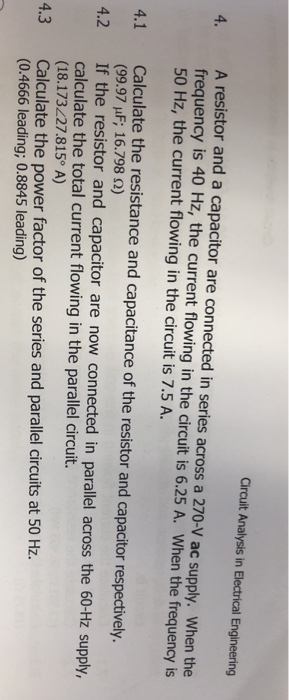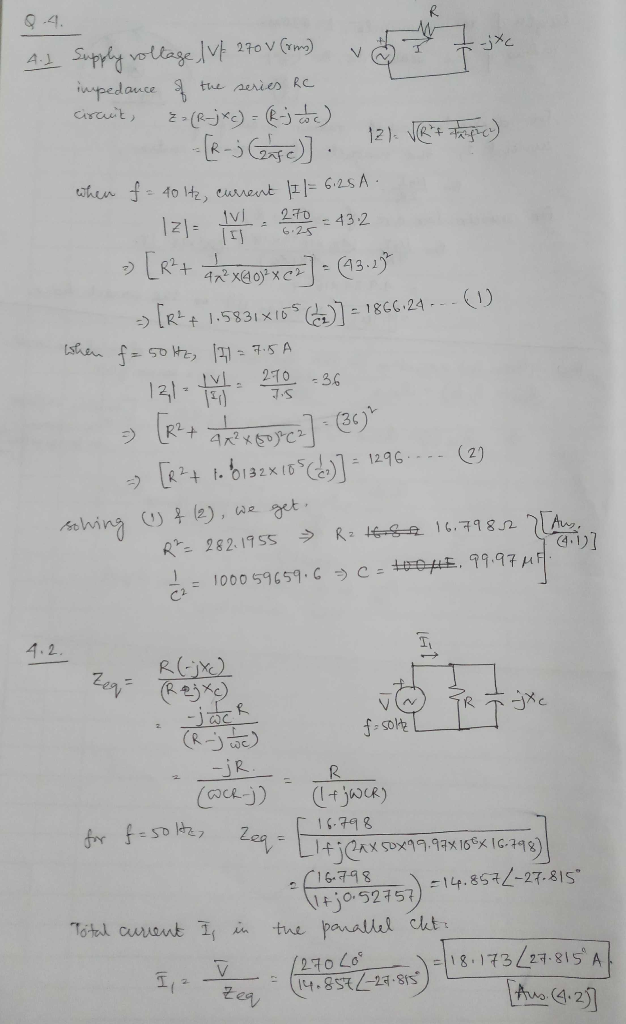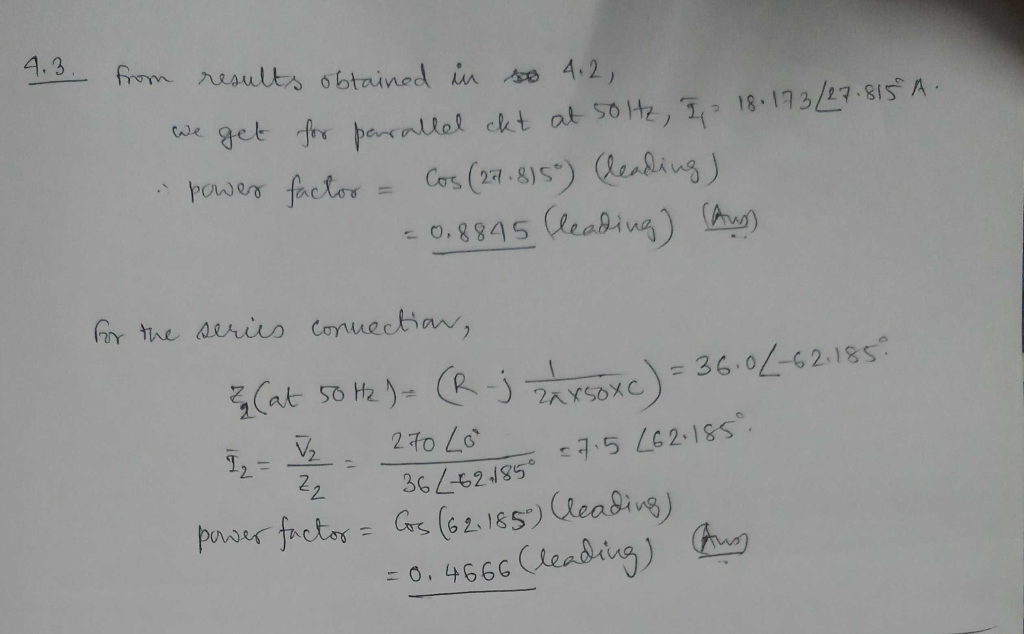#### Earn Coins

Coins can be redeemed for fabulous gifts.

Similar Homework Help Questions
• ### A series combination of a resistor and a capacitor is connected to a 110-V rms, 59.7-Hz...

A series combination of a resistor and a capacitor is connected to a 110-V rms, 59.7-Hz ac source. If the capacitance is 0.860 μF and the rms current in the circuit is 24.2 mA, what is the resistance?

• ### A circuit is constructed with an AC power supply, with a peak voltage of 12 V...

A circuit is constructed with an AC power supply, with a peak voltage of 12 V and a frequency of 50 Hz, connected in series with a 200 Ω resistor, a 300 mH inductor and a 470 μF capacitor. Calculate the peak value of the voltage across the resistor, in V.

• ### A circuit has an ac voltage source and a resistor and capacitor connected in series. There is no inductor.

A circuit has an ac voltage source and a resistor and capacitor connected in series. There is no inductor. The ac voltage source has voltage amplitude 0.900 kV and angular frequency w = 20.0 rad/s. The voltage amplitude across the capacitor is 0.500 kV. The resistor has resistance R= 0.300 kΩ. Part A What is the voltage amplitude across the resistor? Part B What is the capacitance C of the capacitor? Part C Does the source voltage lag or lead the current? Part D What is the average...

• ### In a series circuit, a generator (1300 Hz, 15.0 V) is connected to a 14.0- resistor,...

In a series circuit, a generator (1300 Hz, 15.0 V) is connected to a 14.0- resistor, a 3.90-μF capacitor, and a 5.80-mH inductor. Find the voltages across (a) the resistor, (b) the capacitor, and (c) the inductor. Note: The ac current and voltage are rms values and power is an average value unless indicated otherwise.

• ### (a) A capacitor of capacitance 220 μF is connected in series with a 150 kΩ resistor, a switch and an ammeter. A d.c. power supply of negligible internal resistance is connected to the circuit as show...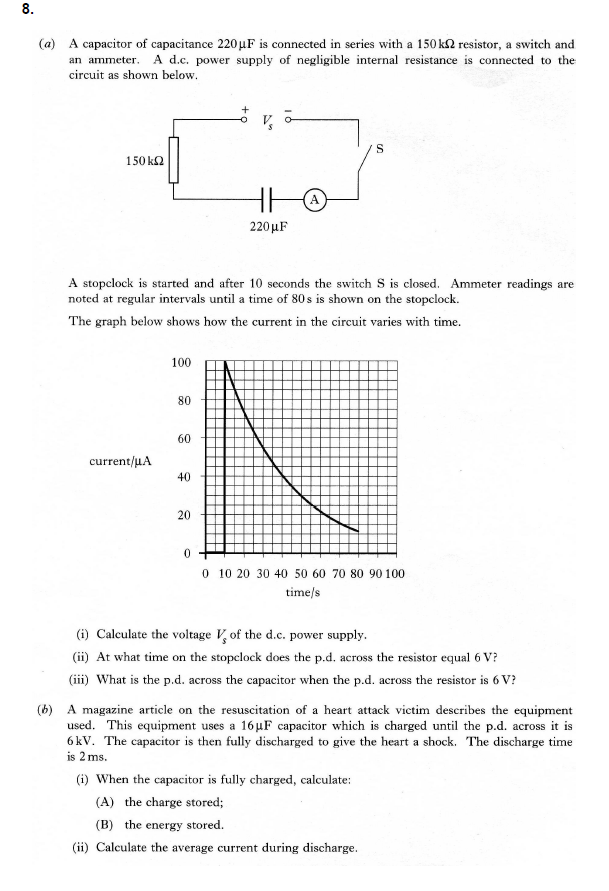(a) A capacitor of capacitance 220 μF is connected in series with a 150 kΩ resistor, a switch and an ammeter. A d.c. power supply of negligible internal resistance is connected to the circuit as shown below 1 50 kΩ 220HF A stopclock is started and after 10 seconds the switch S is closed Ammeter readings are noted at regular intervals until a time of 80s is shown on the stopclock. The graph below shows how the current in the...

• ### A continuous AC voltage with peak voltage of 6 V and frequency f=732398 Hz is connected...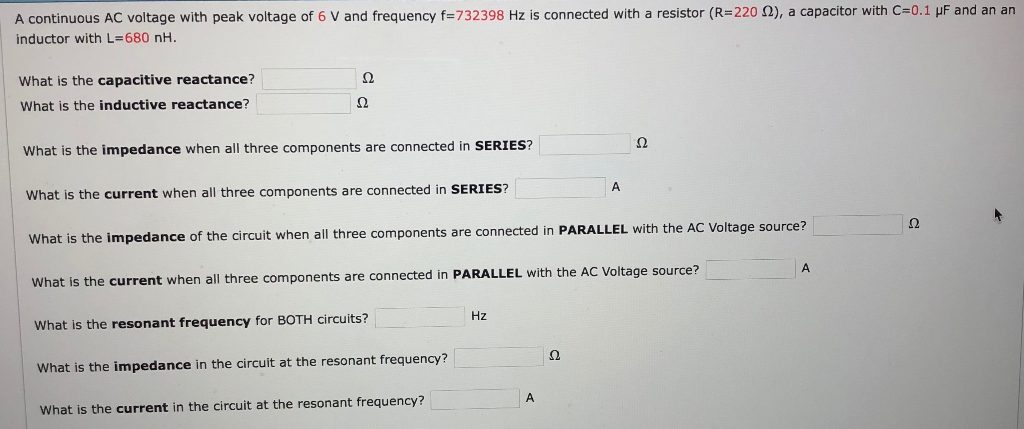A continuous AC voltage with peak voltage of 6 V and frequency f=732398 Hz is connected with a resistor (R-220 Ω), a capacitor with C-0.1 μΕ and an an inductor with L=680 nH. What is the capacitive reactance? What is the inductive reactance? What is the impedance when all three components are connected in SERIES? What is the current when all three components are connected in SERIES? What is the impedance of the circuit when all three components are connected...

• ### A capacitor and a resistor that are in series are initially connected to a power supply...

A capacitor and a resistor that are in series are initially connected to a power supply with 22 volts. The power supply is then cut-off and the capacitor begins to discharge through the resistor. At t = 20 s, the voltage across the capacitor drops to 7 volts. What is the resistance of the resistor, in mega ohms (MΩ) if the capacitance, C = 7 μF ? Please give your answer in two decimal places.

• ### A 3490 - Ω resistor and a 1.7 - μF capacitor are connected in series across...

A 3490 - Ω resistor and a 1.7 - μF capacitor are connected in series across a generator (60.0 Hz, 120 V). Determine the power delivered to the circuit.

• ### An AC source operating at 60-Hz with a maximum voltage of 170 V is connected in series with a resistor (R = 1.2 k Q) and capacitor (C = 2.5 μF).

An AC source operating at 60-Hz with a maximum voltage of 170 V is connected in series with a resistor (R = 1.2 k Q) and capacitor (C = 2.5 μF). a) What is the maximum value of the current in the circuit? b) What is the maximum values of the potential difference across the resistor and capacitor? c) When the current is zero, what are the magnitudes of the potential difference across the resistor, capacitor and AC source? How much charge is...

• ### A 100uF capacitor, a 5H inductor and a 10052 Resistor are connected in series across a...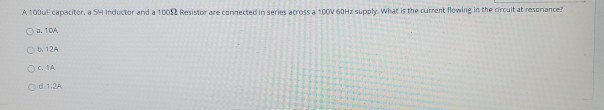A 100uF capacitor, a 5H inductor and a 10052 Resistor are connected in series across a 100V 60Hz supply. What is the current flowing in the circuit at resonance? a. 104 1. 12A OGIA Od 1:24